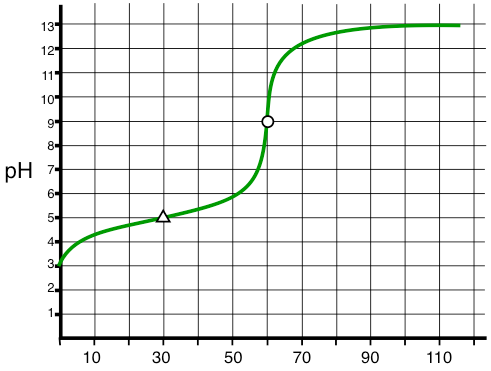# Problem: What is the most likely identity of the species undergoing titration?a. hydrobromic acid (HBr); Ka = 1.08 x 109b. acetic acid (CH3CO2H); Ka = 1.8 x 10-5c. ammonia (NH3); Kb = 1.8 x 10-5d. sodium acetate (NaC2H3O2); Kb = 5.6 x 10-10

###### FREE Expert Solution

From the titration curve:

Equivalence point = 60 mL           pH = 9

Half-equivalence point = 30 mL     pH = 5

91% (47 ratings)###### Problem Details

What is the most likely identity of the species undergoing titration?a. hydrobromic acid (HBr); Ka = 1.08 x 109

b. acetic acid (CH3CO2H); Ka = 1.8 x 10-5

c. ammonia (NH3); Kb = 1.8 x 10-5

d. sodium acetate (NaC2H3O2); Kb = 5.6 x 10-10